# 普林斯顿·META

2023-10-17更新

## 舰船信息

 普林斯顿·META Princeton META プリンストン(META)编号 NO.META026 类型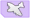轻航 稀有度 ★★☆☆☆精锐 阵营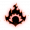META-??? 实装日期 2023年10月01日 相关活动相关活动默认包含该活动的复刻 建造时间 无法建造 档案掉落点 活动掉落点请注意！首次活动、复刻活动、作战档案三者掉落可能会有差异，所以如果没列出作战档案掉落则表示作战档案中不掉落。 其他途径 第十三期基础巡游奖励 营养价值 不可用作强化材料 退役收益 无法退役 强化加成点击查看强化需求 炮击 0 耐久 794 雷击 0 防空 47 航空 66 命中 24 装填 50 机动 16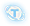+ 12

55+ 25
-
Lv.120+ 18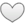耐久 B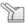防空 B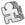机动 C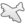航空 A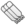雷击 E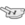炮击 E
 初始→`125级`满破满强化 好感度爱属性 耐久 814→5006 装甲 中型 装填 66→183 炮击 0→0 雷击 0→0 机动 16→86 防空 48→259 航空 67→359 命中 25→102 反潜 17→78 幸运 49 消耗 7→12 航速 32 碧蓝海事局 普林斯顿·META 详细数据等级 120　强化 满　突破 3　好感度 爱综合性能：（不含舰队科技） 耐久 装甲 中型 装填 炮击 雷击 机动 防空 航空 命中 反潜 幸运 消耗 航速
 突破效果 一阶 习得技能【破敌之炬·普林斯顿】/所有战斗机+1/战斗机效率提高5% 二阶 机库容量+1/所有鱼雷机+1/战斗机效率提高10% 三阶 【破敌之炬·普林斯顿】升级/所有舰载机+1/鱼雷机效率提高15%
 满破装备 槽 装备类型 效率 武器数 预装填数 1 战斗机 125% 3 0 2 鱼雷机 125% 3 0 3 防空炮 80% 1 1 4 设备 - - - 5 设备 - - - 6 特殊兵装 - - - 注 水面鱼雷底座+1与航母机库+1并不增加武器数

1 -
2 -
3 -

 技能燃火之磷 自身受到的点燃伤害降低5.0%（15.0%），场上存在被点燃单位时自身造成的伤害提升4.5%（12.0%）；自身装备的舰载机武器命中敌方单位时，有40.0%（70.0%）的概率对命中敌人造成特殊点燃效果，特殊点燃持续10秒隐入雨云 每次执行空袭后，使自身受到的伤害降低5.0%（15.0%），并使自身被侦测条回复速率提升1点/秒，持续8秒，伤害降低效果结束后有40.0%（70.0%）的概率发动一轮航空弹幕Lv.1（Lv.10）破敌之炬·普林斯顿I（II） 【大型作战效果】与精英舰队或BOSS舰队交战时，自身造成的所有伤害提高2.0%（6.0%）
 立绘 通常换装改造誓约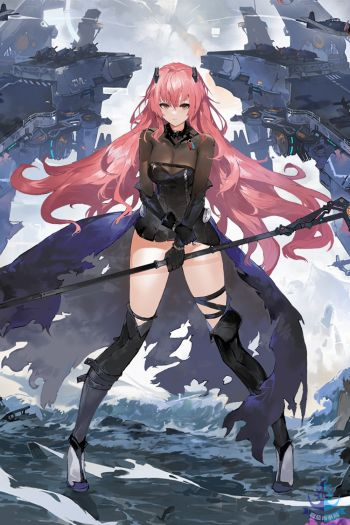角色信息 身份 性格 关键词 持有物 发色 瞳色 萌点 CV 画师 + 微博 - 推特 - PIXIV
 强度评价 技能数据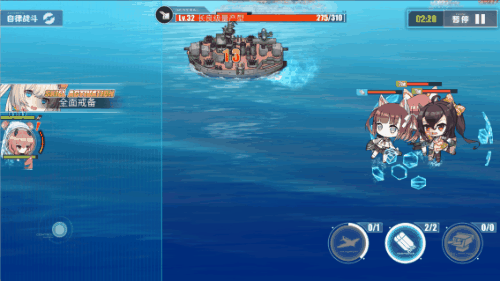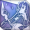隐入雨云航空弹幕： 191×12hit平行雷，对甲补正80% 110% 130%。 备注

## 舰船台词

登录界面 碧蓝航线！ 我是独立级二号舰普林斯顿，曾隶属于与安蒂克斯一同的联合舰队。我不知道为何出现在这里，对这边也并不熟悉……但直觉告诉我，你是值得信任的指挥官，我会听从你的命令。 航空母舰普林斯顿，向指挥官报到……对不起，我现在还有些混乱，方便的话能说明一下现状吗？ 航空母舰普林斯顿，今日也听候你的差遣，指挥官。 “塞壬”？这是它们现在的名字吗？而且为什么我们正与她们为敌？……抱歉，我不应该问这些，只是确实不太明白…… 小、小加加？！我怎么能对萨拉托加前辈用这么亲昵的称呼！还是“萨拉托加前辈”就好。 如果装备能进一步升级的话，就可以在战斗中承担更多的责任了。 为指挥官分忧是秘书舰的职责。请问有什么需要我帮忙的么？ 任务已经完成了。这些奖励刚好可以扩充后勤物资储备。 指挥官，你是不是状态不太好？你也许需要休息…… 指挥官，可以的话，能和我讲讲你知道的，可能和我有关的事么？身为下属本不应该问这些，但我还是想知道，到底发生了什么。 我只记得自己去执行一项特殊的任务，结果和同伴们走散了，被困到了奇怪的地方。终于脱困之后便到了这里，没想到已经过去了这么久…… 直觉告诉我，指挥官是值得信任的人，你也用行动证明了我是对的。请不用顾虑，尽情驱使我的力量为你所用吧。 错过的事无法再弥补，但只要我们能走在正确的道路上，一切就并非毫无意义。指挥官，我相信你所走的路，我也愿意与你一同前行。 这，这是选择了我的意思吗？没想到你会如此重视我……那我就更加不能离开你了。我愿意将自己的全部托付给你，一起向着新的未来前进吧！ 委托组顺利返航了。有没有什么需要我去帮忙的？

## 其它舰船

驱逐 • 埃尔德里奇 • 哈曼II • 杜威 • 艾尔温 • 卡辛改 • 唐斯改 • 格里德利 • 克雷文 • 麦考尔 • 莫里 • 西姆斯改 • 哈曼改 • 本森 • 拉菲改 • 贝利改 • 霍比 • 科尔克 • 弗莱彻 • 拉德福特 • 杰金斯 • 尼古拉斯改 • 贝奇 • 斯坦利 • 富特 • 斯彭斯 • 撒切尔 • 金伯利 • 马拉尼 • 布什 • 黑泽伍德 • 史蒂芬·波特 • 莫里森 • 斯莫利 • 奥利克 • 查尔斯·奥斯本改 • 哈尔西·鲍威尔 • 艾伦·萨姆纳 • 英格拉罕 • 库珀 • 布里斯托尔 • 奥马哈 • 罗利 • 里士满 • 康克德 • 马布尔黑德 • 孟菲斯 • 布鲁克林 • 菲尼克斯 • 博伊西 • 火奴鲁鲁 • 圣路易斯 • 海伦娜改 • 小海伦娜 • 亚特兰大 • 朱诺改 • 圣地亚哥改 • 小圣地亚哥 • 圣胡安 • 里诺 • 克利夫兰 • 小克利夫兰 • 克利夫兰(μ兵装) • 哥伦比亚 • 蒙彼利埃 • 丹佛 • 伯明翰 • 比洛克西 • 休斯敦II • 西雅图 • 彭萨科拉 • 盐湖城 • 北安普敦 • 芝加哥 • 休斯敦 • 波特兰改 • 印第安纳波利斯 • 新奥尔良 • 阿斯托利亚 • 明尼阿波利斯 • 旧金山 • 昆西 • 文森斯 • 威奇塔 • 巴尔的摩 • 巴尔的摩(μ兵装) • 布莱默顿 • 北安普敦II • 安克雷奇 • 内华达改 • 俄克拉荷马改 • 宾夕法尼亚 • 亚利桑那 • 田纳西 • 加利福尼亚 • 科罗拉多 • 马里兰 • 西弗吉尼亚改 • 北卡罗来纳 • 华盛顿 • 南达科他 • 马萨诸塞 • 阿拉巴马 • 佐治亚 • 新泽西 • 奇尔沙治 • 兰利改 • 突击者改 • 长岛改 • 博格改 • 卡萨布兰卡 • 独立改 • 普林斯顿 • 兰利II • 巴丹 • 列克星敦 • 萨拉托加改 • 约克城 • 企业 • 小企业 • 大黄蜂 • 胡蜂 • 埃塞克斯 • 约克城II • 无畏 • 大黄蜂II • 提康德罗加 • 邦克山 • 香格里拉 • 鹦鹉螺 • 大青花鱼 • 大青花鱼(μ兵装) • 蓝鳃鱼 • 棘鳍 • 鲦鱼 • 射水鱼 • 女灶神
驱逐 • 吸血鬼 • 女将改 • 阿卡司塔改 • 热心改 • 小猎兔犬 • 大斗犬 • 彗星改 • 小天鹅改 • 新月改 • 回声 • 命运女神改 • 狐提改 • 萤火虫 • 格伦维尔 • 勇敢 • 英雄 • 猎人 • 伊卡洛斯 • 爱斯基摩人 • 贾维斯 • 天后 • 雅努斯 • 标枪改 • 泽西 • 丘比特 • 火枪手 • 无敌 • 库拉索改 • 杓鹬改 • 进取 • 利安得改 • 阿基里斯改 • 阿贾克斯改 • 阿瑞托莎 • 加拉蒂亚 • 佩内洛珀 • 欧若拉 • 纽卡斯尔改 • 南安普顿 • 谢菲尔德 • 谢菲尔德(μ兵装) • 格拉斯哥 • 格罗斯特 • 曼彻斯特 • 爱丁堡 • 贝尔法斯特 • 小贝法 • 黛朵 • 黛朵(μ兵装) • 赫敏 • 天狼星 • 卡律布狄斯 • 斯库拉 • 司战女神 • 黑太子 • 斐济 • 牙买加 • 确捷 • 海王星 • 普利茅斯 • 肯特 • 萨福克改 • 伦敦改 • 苏塞克斯 • 什罗普郡改 • 诺福克 • 多塞特郡 • 约克改 • 埃克塞特改 • 柴郡 • 小柴郡 • 德雷克 • 声望 • 小声望 • 反击 • 胡德 • 复仇 • 皇家橡树 • 伊丽莎白女王 • 厌战改 • 英勇 • 纳尔逊改 • 罗德尼 • 英王乔治五世 • 威尔士亲王 • 约克公爵 • 豪 • 君主 • 前卫 • 百眼巨人 • 竞技神改 • 追赶者 • 独角兽改 • 英仙座 • 忒修斯 • 半人马 • 阿尔比恩 • 鹰 • 光荣 • 皇家方舟改 • 光辉 • 小光辉 • 光辉(μ兵装) • 胜利 • 可畏 • 小可畏 • 不挠 • 怨仇 • 黑暗界 • 恐怖 • 阿贝克隆比
驱逐 • 神风改 • 松风改 • 旗风 • 追风 • 朝凪 • 睦月改 • 如月改 • 卯月 • 水无月 • 文月 • 长月 • 三日月 • 吹雪 • 白雪 • 深雪 • 浦波 • 绫波改 • 晓 • 响 • 雷 • 电 • 初春改 • 若叶 • 初霜改 • 有明改 • 夕暮改 • 白露 • 时雨改 • 夕立改 • 海风 • 山风 • 江风 • 朝潮 • 大潮 • 满潮 • 荒潮 • 霞改 • 阳炎改 • 不知火改 • 黑潮 • 亲潮 • 雪风 • 浦风 • 矶风 • 滨风改 • 谷风改 • 野分 • 风云 • 长波 • 卷波 • 清波 • 凉月 • 初月 • 新月 • 若月 • 春月 • 宵月 • 花月 • 北风 • 岛风 • 长良 • 五十铃改 • 名取 • 由良 • 鬼怒改 • 阿武隈改 • 川内改 • 神通改 • 那珂 • 夕张改 • 最上改 • 三隈 • 阿贺野 • 能代 • 酒匂 • 四万十 • 古鹰改 • 加古改 • 青叶 • 衣笠 • 妙高 • 那智改 • 足柄 • 羽黑 • 高雄 • 爱宕 • 摩耶 • 鸟海 • 铃谷 • 熊野 • 筑摩 • 伊吹 • 云仙 • 吾妻 • 金刚 • 比叡 • 小比叡 • 榛名 • 雾岛 • 天城 • 小天城 • 三笠 • 扶桑改 • 山城改 • 伊势改 • 日向改 • 长门 • 陆奥 • 加贺 • 土佐 • 纪伊 • 尾张 • 骏河 • 出云 • 武藏 • 凤翔 • 龙骧 • 祥凤改 • 龙凤 • 千岁 • 千代田 • 飞鹰 • 隼鹰 • 赤城 • 加贺 • 小赤城 • 赤城(μ兵装) • 苍龙改 • 飞龙改 • 翔鹤 • 瑞鹤 • 大凤 • 大凤(μ兵装) • 小大凤 • 葛城 • 信浓 • 白龙 • 伊168 • 伊19 • 伊25 • 伊26 • 伊56 • 伊58 • 伊13 • 明石 • 樫野
驱逐 • Z1改 • Z2 • Z16 • Z18 • Z19 • Z20 • Z21 • Z23改 • Z24 • Z25 • Z26 • Z28 • Z35 • Z36 • Z46 • 奥托·冯·阿尔文斯莱本 • 菲利克斯·舒尔茨 • 埃姆登 • 埃尔宾 • 柯尼斯堡 • 卡尔斯鲁厄改 • 科隆改 • 莱比锡改 • 纽伦堡 • 马格德堡 • 雷根斯堡 • 美因茨 • 约克 • 德意志 • 斯佩伯爵海军上将 • 小斯佩 • 海因里希亲王 • 阿达尔伯特亲王 • 希佩尔海军上将 • 希佩尔海军上将(μ兵装) • 布吕歇尔 • 欧根亲王 • 小欧根 • 罗恩 • 罗恩(μ兵装) • 兴登堡 • 埃吉尔 • 塞德利茨 • 吕佐夫 • 鲁普雷希特亲王 • 沙恩霍斯特 • 格奈森瑙 • 奥丁 • 布伦希尔德 • 图林根 • 俾斯麦 • 俾斯麦Zwei • 提尔比茨 • 腓特烈大帝 • 乌尔里希·冯·胡滕 • 亚德 • 易北 • 威悉 • 齐柏林伯爵 • 小齐柏林 • 彼得·史特拉塞 • 奥古斯特·冯·帕塞瓦尔 • U-47 • U-73 • U-101 • U-81 • U-96 • U-410 • U-556 • U-557 • U-1206 • U-37 • U-110 • U-522
驱逐 • 鞍山改 • 抚顺 • 长春改 • 太原 • 海天 • 海圻 • 肇和 • 应瑞 • 逸仙改 • 宁海改 • 平海改 • 哈尔滨 • 镇海 • 华甲 • 定安
驱逐 • 西北风 • 西南风 • 阿尔弗雷多·奥里亚尼 • 文琴佐·焦贝蒂 • 龙骑兵 • 尼科洛索·达雷科 • 埃曼努埃尔·佩萨格诺 • 阿蒂利奥·雷戈洛 • 庞培·马格诺 • 阿布鲁齐公爵 • 朱塞佩·加里波第 • 特伦托 • 的里雅斯特 • 扎拉 • 戈里齐亚 • 波拉 • 博尔扎诺 • 加富尔伯爵 • 朱利奥·凯撒 • 安德烈亚·多利亚 • 维托里奥·维内托 • 利托里奥 • 罗马 • 马可·波罗 • 天鹰 • 帝国 • 托里拆利 • 莱昂纳多·达·芬奇
驱逐 • 威严 • 神速 • 雷鸣 • 洪亮 • 灵敏 • 明斯克 • 基辅 • 塔什干 • 塔什干(μ兵装) • 阿芙乐尔 • 水星纪念改 • 摩尔曼斯克 • 基洛夫 • 伏罗希洛夫 • 恰巴耶夫 • 古比雪夫 • 塔林 • 库尔斯克 • 喀琅施塔得 • 甘古特 • 塞瓦斯托波尔 • 阿尔汉格尔斯克 • 苏维埃贝拉罗斯 • 苏维埃罗西亚 • 契卡洛夫 • 伏尔加
驱逐 • 福尔班改 • 鲁莽 • 倔强 • 马耶·布雷泽 • 可怖 • 凯旋 • 圣女贞德 • 埃米尔·贝尔汀改 • 吉尚 • 絮弗伦 • 路易九世 • 布雷斯特 • 里昂 • 黎塞留 • 香槟 • 贝亚恩 • 伴尔维 • 絮库夫
驱逐 • 勒马尔改 • 沃克兰 • 凯尔圣 • 塔尔图 • 恶毒 • 恶毒(μ兵装) • 不屈 • 拉·加利索尼埃 • 马赛曲 • 福煦 • 阿尔及利亚 • 敦刻尔克 • 让·巴尔 • 克莱蒙梭 • 加斯科涅 • 加斯科涅(μ兵装) • 弗兰德尔 • 霞飞
驱逐 • 泛用型布里 • 试作型布里MKII • 特装型布里MKIII
第一期 • 克利夫兰(μ兵装) • 谢菲尔德(μ兵装) • 赤城(μ兵装) • 希佩尔海军上将(μ兵装) • 加斯科涅(μ兵装) • 巴尔的摩(μ兵装) • 大青花鱼(μ兵装) • 黛朵(μ兵装) • 光辉(μ兵装) • 塔什干(μ兵装) • 大凤(μ兵装) • 罗恩(μ兵装) • 恶毒(μ兵装)
风帆S • 皇家财富号 • 玛丽·西莱斯特号 • 维达号 • 金鹿号 • 加里冒险号 • 圣马丁号
META • 普林斯顿·META • 海伦娜·META • 谢菲尔德·META • 沙恩霍斯特·META • 反击·META • 声望·META • 扶桑·META • 飞鹰·META • 隼鹰·META • 飞龙·META • 皇家方舟·META • 旗风·META • 孟菲斯·META • 神通·META • 亚利桑那·META • 女灶神·META • 猎人·META • 命运女神·META • 拉·加利索尼埃·META • 阿尔及利亚·META • 格奈森瑙·META • 伊丽莎白女王·META • 黑暗界·META • 基洛夫·META • 特伦托·META • 山城·META • 苍龙·META • U-556·META
驱逐 • 布兰 • 群白之心 • 涅普顿 • 绀紫之心 • 诺瓦露 • 圣黑之心 • 贝露 • 翡绿之心
驱逐 • 22 • 33
驱逐 • 猫音 • 露露缇耶 • 久远 • 乌璐露 • 萨拉娜 • 芙米露露
驱逐 • 绊爱 • 绊爱·Elegant • 绊爱·SuperGamer • 绊爱·Anniversary
驱逐 • 玛莉萝丝 • 海咲 • 莫妮卡 • 霞 • 环 • 穗香 • 凪咲 • 露娜 • 女天狗
轻巡 • 天海春香 • 三浦梓 • 水濑伊织 • 如月千早 • 双海亚美 • 双海真美 • 秋月律子
轻巡 • 宝多六花 • 莲 • 奈美子 • 南梦芽 • 新条茜 • 貉 • 第二代 • 飞鸟川千濑 • 公主
轻巡 • 莱莎琳·斯托特 • 莉拉·德西亚斯 • 卡菈·伊迪亚斯 • 帕特莉夏·阿贝尔海姆 • 科洛蒂娅·巴兰茨 • 赛莉·古劳斯
轻巡 • 飞鸟 • 斑鸠 • 夕烧 • 雪不归 • 紫 • 雪泉 • 焰